• ## sklearn决策树

千次阅读 2018-04-22 03:00:53
对信息做了度量，但是追根究底，度量却不是目的。手段不是目的，这是缺月梧桐中的名句。有多少迷失在路上的人，应该时刻的回想起自己的初衷。对信息有了评判标准以后，关键的才开始。以这个为依据，我们能做些什么，...
对信息做了度量，但是追根究底，度量却不是目的。手段不是目的，这是缺月梧桐中的名句。有多少迷失在路上的人，应该时刻的回想起自己的初衷。对信息有了评判标准以后，关键的才开始。以这个为依据，我们能做些什么，怎么去做？对信息做了度量之后，就可以有对比，对比的结果指引了方向，损失函数什么的也有了度量。根据方向，不断前行，这是我们的价值所在。和机器不同在于1. 人的精神粮食指引方向，方向不明确；朝方向前进，前进的方式也不明确2. 机器只会做明摆着的事情，方向和前进必然要明确决策树就是如此，不断的if....else，做的事，判断的标准必须十分明确，然后得出实在的结论，从不随心所欲。决策树模型建立    1. 机器识别的数据    说到底，机器是死物。理解不了概念，始终只能操作数值。灵智的语言，概念，它不会去懂得。当然，也无需懂得。我们不会狗的语言，我们要它们靠近的时候，也不必与它们沟通----有肉就行。所以，机器可以处理，但不会习得，但我们也只是需要结果而已。只要它让我们分不清机器还是人，那就是智能。我们智能化机器，为我们所用；机器也可以数值化人类，从而能够交流。    类比于身高和体重的计算，我们可以有一个精密的计算公式，机器对这种数值计算式天生的能手，迅速的就能给出结果。但是男女有差异，加上这一判别之后，人类计算速率依旧不变，机器却无法处理。因为机器不分男女，只认0,1。    但是机器仍然能够认识3甚至更多的数，那需要一种转换的方式，一种数值化的方式让机器可以理解。我们可以用0表示男，用1表示女，机器就能够识别了。十分简单，却并不方便快捷。因为机器人认识数值，但值是有大小的。对于类型判别一类，数值表示法并不总是能够成功。    概念性的东西，不能通过单一的数值来进行表示，原因在于数值的大小会让影响的力度和粒度发生变化。我们必须保证它只是做了简单的逻辑替换，而不是更换了整个逻辑体系。所以男就是男，女就是女，两者之间有且只有一种联系，那就是性别的描述。这个量，叫做性别，它的取值有两个，男和女，他们的作用是同一的，只是分支的判断而已。所以，这种分类的枚举，机器该怎么才能进行准确的识别？单一的数值的确能够区分，但是空间除了0和1还会有3和更多的取值，性别可没多样化到这种程度。    维度。引入维度可以更好的描述此类问题。我们用两个值的组合来描述它，这是两个数，却是一个值。这个值是性别，其中不是男就是女，而替换为数值，就仅仅是是或者是不是，也就是0，或者1。男[1, 0]，女[0, 1]。这样，男女的判别就融合在一个完整的逻辑中，采用0或者1，来启用或者屏蔽部分逻辑从而达到分支的判别。    可能产生的歧义是[1,1]或者[0,0]这种奇葩，这种向量有两种可能，对于有四分类的，区别于[0,1]和[1,0]，表述的是其他两种状态，是两个数位共同决定的一个值。另一种情况，就是错误的输入了，既男又女？不男不女？如果是类别区分，只能有一个被启用，其他的都是错误输入。    经过这种转换，概念性的东西就可以被数值化，被机器所能够理解。老年，中年，青年，少年，也不过是转化成了一个四维的数值。就这样，人类的概念才得以被机器所接受和处理。概念性的东西，都是这样被转化，是一种技巧，却也是一种固定的方式。    2. 数据格式化    数据数值化，就是这样了。想要机器能够接受，数值化必不可少。手工也可以做，不过机器做这种事更加擅长。它不可以直接的解析，却也可以进行转换。我们就尽情的剥削他们，让他们自己先转换，然后再自己解析。   加载数据datafile = open('test.csv', 'r')
data = table[1:]
labels = []
dataSet = []

for line in data:
temp = {}
for index in range(1, len(line) - 1):
dataSet.append(temp)
labels.append(line[-1])    python自带的csv，标签和数据分开。    格式化数据dvt = DictVectorizer()
X = dvt.fit_transform(dataSet).toarray()    格式化标签lb = preprocessing.LabelBinarizer()
Y = lb.fit_transform(labels)这两个东西，需要sklearn的库from sklearn.feature_extraction import DictVectorizer
import csv
from sklearn import preprocessing    3. 模型创建    模型创建的过程呢，就是这样model = tree.DecisionTreeClassifier(criterion='entropy')
model = model.fit(X, Y)需要from sklearn import tree    不用怀疑，就是这点了，因为是工具，全部被封装了。后台的全部原理就是信息的一套理论，判别依据entropy就是讨论过的信息熵。简单的函数底层实现的就是之前的全部细节。除了ID3还有其他衡量标准，那就自己学吧。    毕竟是工具，原理需要自己去学习，但是工具只要会用，就会有结果。细节全部隐藏。python很好用，不过想明白可不简单。    还有一点，关于剪枝。过于深入的枝叶判断很没必要    1. 数据遍历消耗大    2. 数据过拟合    所以决策树一般要进行剪枝----必要的话。这东西也被封装了，想要操作更多功能，看手册吧。图像表示    1. 逻辑文件保存    模型建立好了，就可以把它保存下来，下次也可以用。更好的进行迁移。with open('result.dot', 'w') as file:
file = tree.export_graphviz(model, feature_names=dvt.get_feature_names(), out_file=file)    2. 图像表示既然是逻辑图，我们就可以把它图像表示。导出的dot文件可以经过graphviz生成形象的逻辑表示图。命令行运行 dot -Tpgn result.dot -o test.png  就会生成逻辑图了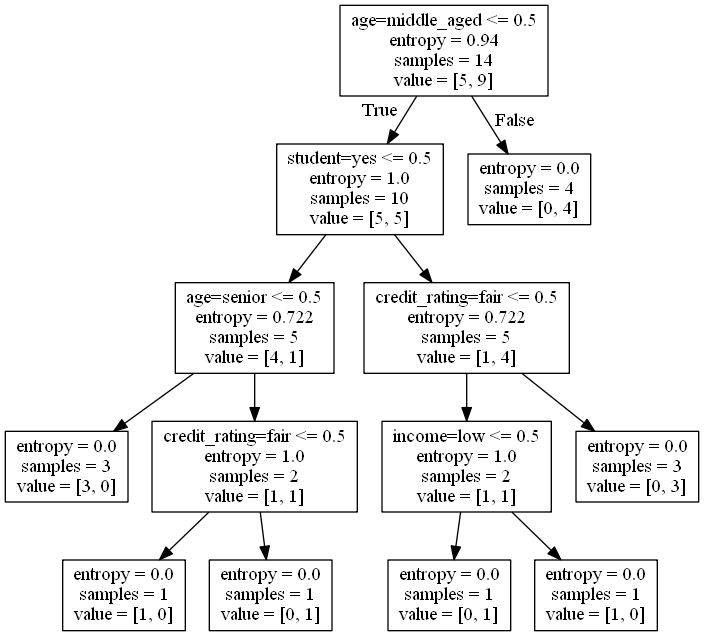索引判别    1. 数据格式化    同样的道理，测试数据也是你也要进行格式化，格式对不上的话，怎么跑流程。其中的新数据，不是原始数据，它同样的经过了DictVectorizer进行过了格式化。简而言之，你建模的输入是什么格式，测试的输入也必然是什么格式。    2. 模型判断result = model.predict(newOne)测试数据格式化之后，模型predict就直接让它进去自己路由了，就只是输出一个结果，这多么的方便快捷。    1. 机器自动格式化数据    2. 机器自动建模    3. 机器自动判定把解析文件部分也写成程序，拖入文件，然后就可以直接进行数据的分析了。真特么的懒，但是真的很爽快。 不过，这里有一个缺陷，就是你的树必须完整，你逻辑必须覆盖全面。如果有一天一个不男不女的家伙来了，你就遭殃了。所以，测试的数据和训练的数据格式和取值范围必须一致，做到覆盖完全，如果逻辑不完全的话，遇见死路就没办法了。完整代码from sklearn.feature_extraction import DictVectorizer
import csv
from sklearn import preprocessing
from sklearn import tree
# 数据输入
datafile = open('test.csv', 'r')
data = table[1:]
labels = []
dataSet = []

for line in data:
temp = {}
for index in range(1, len(line) - 1):
dataSet.append(temp)
labels.append(line[-1])
# 数据格式化
dvt = DictVectorizer()
X = dvt.fit_transform(dataSet).toarray()
lb = preprocessing.LabelBinarizer()
Y = lb.fit_transform(labels)

# 建模
model = tree.DecisionTreeClassifier(criterion='entropy')
# 训练
model = model.fit(X, Y)

# 保存
with open('result.dot', 'w') as file:
file = tree.export_graphviz(model, feature_names=dvt.get_feature_names(), out_file=file)

# 取数
newOne = X[0, :]
# 修改数据校验结果
newOne = 1
newOne = 0

newOne = [newOne]
# 校验
result = model.predict(newOne)
print(result)练手资源:https://download.csdn.net/download/wait_for_eva/10365738    
展开全文• ## sklearn决策树

千次阅读 2018-01-18 15:49:51
sklearn 决策树
决策树

简介

决策树与SVM一样，都是非常通用的机器学习算法，可以用于分类或者回归问题，以及多输出问题
决策树是之后的集成方法：随机森林的一个基础模块，RF是将生成很多决策树，并进行模型预测
决策树对于数据预处理的要求较低，它的节点主要是条件语句，因此不需要对数据对标准化或者归一化操作。
对于决策树的结果，其特征的重要性程度是可以解释的，是white box模型。而像RF和NN这些算法，都是黑盒模型
训练和可视化

直接利用sklearn中的DecisionTreeClassifier就可以实现基于决策树的分类，如果要对分类结果进行可视化，则需要用到graphviz软件
判别

采用gini纯度来对节点的分类能力进行判断 Gi=1−∑k=1np2i,k<!--//--><![CDATA[//><!--
{G_i} = 1 - \sum\limits_{k = 1}^n {p_{i,k}^2}
//--><!]]>pi,k<!--//--><![CDATA[//><!--
p_{i,k}
//--><!]]>是标签k的第i个节点中，属于类别k的训练样本数
sklearn中使用CART算法进行决策树的分类，每个不是叶子的节点都有2个子节点
估计类的概率

决策树会估计样本属于类别的概率，最大概率所在的类别就是样本的类别
CART(Classifcation And Regression Tree)算法

CART算法的原理：挑选出一个特征k<!--//--><![CDATA[//><!--
k
//--><!]]>和一个阈值tk<!--//--><![CDATA[//><!--
t_k
//--><!]]>，将这个子集下的所有样本分为两类，对于特定的节点，在每次的分类过程中，代价函数(我们需要最小化的函数)为 J(k,tk)=mleftmGleft+mrightmGright<!--//--><![CDATA[//><!--
J(k,{t_k}) = \frac{{{m_{left}}}}{m}{G_{left}} + \frac{{{m_{right}}}}{m}{G_{right}}
//--><!]]>Gleft/right<!--//--><![CDATA[//><!--
G_{left/right}
//--><!]]>是左右子集的Gini系数，用于检测子节点的纯度，mleft/right<!--//--><![CDATA[//><!--
m_{left/right}
//--><!]]>是左右子集的样本个数
算法使用相同的逻辑将子集进行分类，直到树到达最大的深度或者找不到一个可以降低纯度的分割方法，还有一些其他的超参数用于停止算法的迭代，如下 min_samples_split：子节点小于这个数，就不会再进行分割
min_samples_leaf：叶子的样本个数小于这个数，就不会进行此次分割
min_weight_fraction_leaf：分割后，叶子的样本比例小于这个数，就不会进行此次分割
max_leaf_nodes：最大的叶子节点个数
CART算法是贪婪算法，它在每一步只搜索当前的最优解，不能保证最终得到的是全局最优解
平均来说，CART算法的时间复杂度为:O(n⋅m⋅log(m))<!--//--><![CDATA[//><!--
O(n \cdot m \cdot log(m))
//--><!]]>
除了Gini纯度作为代价函数，也可以设置熵纯度( entropy impurity)，熵越小，表示系统静止或有序，表示一个能量较低的状态
某个节点的熵为 Hi=−∑k=1,pi,k≠0npi,klog(pi,k)<!--//--><![CDATA[//><!--
{H_i} =  - \sum\limits_{k = 1,{p_{i,k}} \ne 0}^n {{p_{i,k}}\log ({p_{i,k}})}
//--><!]]>
大部分情况下，Gini纯度与熵纯度得到的树都类似，Gini纯度的计算速度比熵纯度要稍微快一点；相对于Gini纯度，熵纯度作为代价函数得到的树会更加平衡
正则化超参数

决策树不对训练数据做出什么假设，即比如说数据符合线性模型等，因此常常会对训练数据过拟合。这样的模型也可以成为非参数模型。因为参数个数是由训练数据确定的。而对于参数化模型来说，其参数个数是预确定的，模型的自由度有限，因此也就降低了过拟合的风险。
为了降低决策树过拟合的危险，可以引入上面提到的一些参数
import os
import numpy as np
from sklearn.tree import DecisionTreeClassifier
from sklearn.tree import export_graphviz
X = iris.data[:,2:]
y = iris.target

tree_clf = DecisionTreeClassifier( max_depth=2 )
tree_clf.fit( X, y )

# 导出图
# export_graphviz( tree_clf,
#                  out_file="images/decision_trees/tree_clf.dot",
#                  feature_names=iris.feature_names[2:],
#                  class_names=iris.target_names,
#                 rounded=True,
#                 filled=True
#                 )
print( tree_clf.predict_proba([[5, 1.5]]) )
print(tree_clf.predict([[5, 1.5]]))

[[ 0.          0.33333333  0.66666667]]



%matplotlib inline
import matplotlib.pyplot as plt
from matplotlib.colors import ListedColormap

def plot_decision_boundary(clf, X, y, axes=[0, 7.5, 0, 3], iris=True, legend=False, plot_training=True):
x1s = np.linspace(axes, axes, 100)
x2s = np.linspace(axes, axes, 100)
x1, x2 = np.meshgrid(x1s, x2s)
X_new = np.c_[x1.ravel(), x2.ravel()]
y_pred = clf.predict(X_new).reshape(x1.shape)
custom_cmap = ListedColormap(['#fafab0','#9898ff','#a0faa0'])
plt.contourf(x1, x2, y_pred, alpha=0.3, cmap=custom_cmap, linewidth=10)
if not iris:
custom_cmap2 = ListedColormap(['#7d7d58','#4c4c7f','#507d50'])
plt.contour(x1, x2, y_pred, cmap=custom_cmap2, alpha=0.8)
if plot_training:
plt.plot(X[:, 0][y==0], X[:, 1][y==0], "yo", label="Iris-Setosa")
plt.plot(X[:, 0][y==1], X[:, 1][y==1], "bs", label="Iris-Versicolor")
plt.plot(X[:, 0][y==2], X[:, 1][y==2], "g^", label="Iris-Virginica")
plt.axis(axes)
if iris:
plt.xlabel("Petal length", fontsize=14)
plt.ylabel("Petal width", fontsize=14)
else:
plt.xlabel(r"$x_1$", fontsize=18)
plt.ylabel(r"$x_2$", fontsize=18, rotation=0)
if legend:
plt.legend(loc="lower right", fontsize=14)
return

plt.figure(figsize=(5, 3))
plot_decision_boundary(tree_clf, X, y)
plt.show()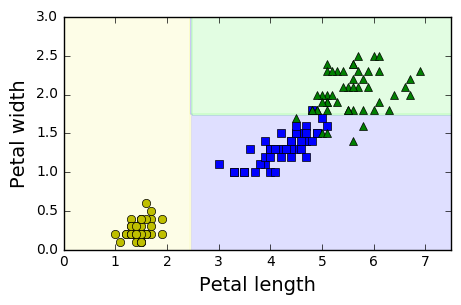# 添加的这个超参数可以有效地降低模型的过拟合程度
from sklearn.datasets import make_moons
Xm, ym = make_moons(n_samples=100, noise=0.25, random_state=53)

deep_tree_clf1 = DecisionTreeClassifier(random_state=42)
deep_tree_clf2 = DecisionTreeClassifier(min_samples_leaf=4, random_state=42)
deep_tree_clf1.fit(Xm, ym)
deep_tree_clf2.fit(Xm, ym)

plt.figure(figsize=(8, 3))
plt.subplot(121)
plot_decision_boundary(deep_tree_clf1, Xm, ym, axes=[-1.5, 2.5, -1, 1.5], iris=False)
plt.title("No restrictions", fontsize=16)
plt.subplot(122)
plot_decision_boundary(deep_tree_clf2, Xm, ym, axes=[-1.5, 2.5, -1, 1.5], iris=False)
plt.title("min_samples_leaf = {}".format(deep_tree_clf2.min_samples_leaf), fontsize=14)

plt.show()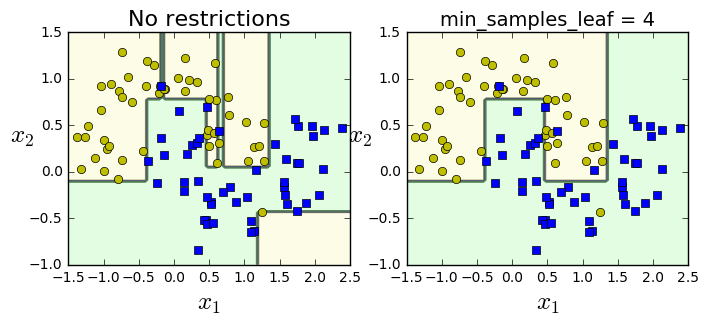回归问题

与分类问题类似，对于回归问题，首先需要构建一棵树，需要求某个样本的值的时候，首先找到该样本所在的叶子节点，然后对该节点上的所有值取平均，就是该样本的平均值，每个节点都有对应的mse
回归问题的代价函数是 J(k,tk)=mleftmMSEleft+mrightmMSErightwhere⎧⎩⎨⎪⎪⎪⎪⎪⎪⎪⎪MSEnode=∑i∈node(y^node−y(i))2y^node=1mnode∑i∈nodey(i)<!--//--><![CDATA[//><!--
\begin{array}{l}
J(k,{t_k}) = \frac{{{m_{left}}}}{m}MS{E_{left}} + \frac{{{m_{right}}}}{m}MS{E_{right}}\\
where & \left\{ \begin{array}{l}
MS{E_{node}} = \sum\limits_{i \in node} {{{({{\hat y}_{node}} - {y^{(i)}})}^2}} \\
{{\hat y}_{node}} = \frac{1}{{{m_{node}}}}\sum\limits_{i \in node} {{y^{(i)}}}
\end{array} \right.
\end{array}
//--><!]]>
如果没有一些正则化参数，决策树回归模型也很容易发生过拟合
from sklearn.tree import DecisionTreeRegressor

np.random.seed(42)
m = 200
X = np.random.rand(m, 1)
y = 4 * (X - 0.5) ** 2
y = y + np.random.randn(m, 1) / 10

from sklearn.tree import DecisionTreeRegressor

tree_reg = DecisionTreeRegressor(max_depth=2, random_state=42)
tree_reg.fit(X, y)

tree_reg1 = DecisionTreeRegressor(random_state=42)
tree_reg2 = DecisionTreeRegressor(random_state=42, min_samples_leaf=10)
tree_reg1.fit(X, y)
tree_reg2.fit(X, y)

def plot_regression_predictions(tree_reg, X, y, axes=[0, 1, -0.2, 1], ylabel="$y$"):
x1 = np.linspace(axes, axes, 500).reshape(-1, 1)
y_pred = tree_reg.predict(x1)
plt.axis(axes)
plt.xlabel("$x_1$", fontsize=18)
if ylabel:
plt.ylabel(ylabel, fontsize=18, rotation=0)
plt.plot(X, y, "b.")
plt.plot(x1, y_pred, "r.-", linewidth=2, label=r"$\hat{y}$")

plt.figure(figsize=(8, 3))
plt.subplot(121)
plot_regression_predictions(tree_reg1, X, y)
plt.title("no restrictions")
plt.subplot(122)
plot_regression_predictions(tree_reg2, X, y, ylabel=None)
plt.title("min sample leaf num : 10")
plt.show()一些缺点

不稳定：决策树的决策边界关于特征的方向是正交的，因此模型对于训练集的旋转十分敏感，可以采用PCA对数据进行预处理，避免训练集的方向对模型结果的影响
决策树对训练集数据十分敏感，训练集稍微修改一下，决策树形状都可能发生很大的变化

展开全文机器学习 python
• 1. 决策树 重要概念： 根节点：没有进边，有出边。包含最初的，针对特征的提问 中间节点：既有进边也有出边，进边只有一条，出边可以有很多条。都是针对特征的提问。 叶子节点：有进边，没有出边，每个叶子节点都...
文章目录1. 决策树1.1 sklearn中的决策树2. 分类树DecisionTreeClassifier2.1 重要参数2.1.1 criterion2.1.2 random_state & spliter2.1.3 剪枝参数2.1.4 目标权重参数2.2 重要属性和接口2.3 小总结：3 回归树DecisionTreeRegressor3.1 重要参数、属性以及接口3.2 示例：一维回归的图像绘制4 示例：泰坦尼克号幸存者的预测4.1 数据总览4.2 缺失值处理方法4.3 分析数据关系(1) 性别与是否生存的关系 Sex(2) 船舱等级和生存与否的关系 Pclass(3) 年龄与存活与否的关系 Age(4) 称呼与存活与否的关系 Name(5) 有无兄弟姐妹和存活与否的关系 SibSp(6) 有无父母子女和存活与否的关系 Parch(7) 亲友的人数和存活与否的关系 SibSp & Parch(8) 票价分布和存活与否的关系 Fare(9) 船舱类型和存活与否的关系 Cabin(10) 港口和存活与否的关系 Embarked(11) 其他可能和存活与否有关系的特征4.4 变量转换定性(Qualitative)转换：定量(Quantitative)转换：4.5. 特征工程
sklearn的基本建模流程

实例化：建立评估模型对象
通过模型接口训练模型
通过模型接口提取需要的信息

1. 决策树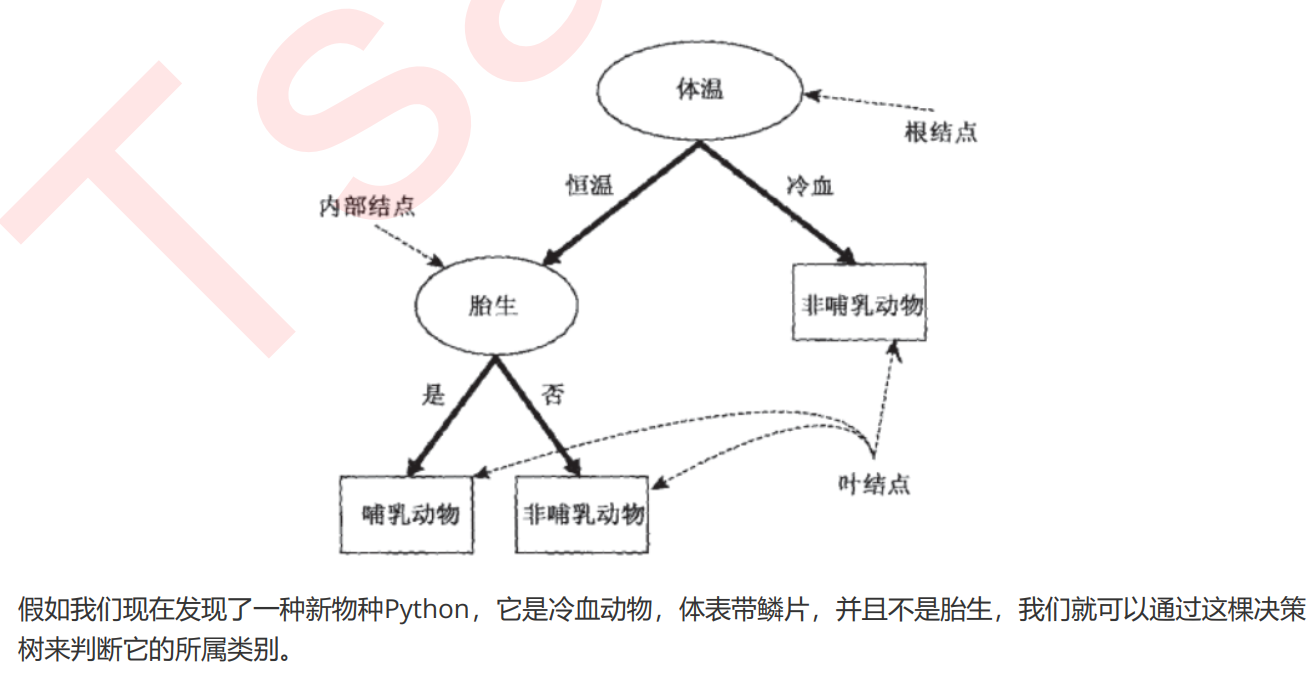重要概念：

根节点：没有进边，有出边。包含最初的，针对特征的提问
中间节点：既有进边也有出边，进边只有一条，出边可以有很多条。都是针对特征的提问。
叶子节点：有进边，没有出边，每个叶子节点都是一个类别标签。
子节点和父节点：在两个相连的节点中，更接近根节点的是父节点，另一个是子节点

决策树算法的核心：

如何从数据表现中发现最佳节点和最佳分枝？
如何让决策树停止生长，防止过拟合

1.1 sklearn中的决策树

模块 sklearn.tree

模块
功能

tree.DecisionTreeClassifier
分类树

tree.DecisiionTreeRegressor
回归树

tree.export_graphviz
生成决策树图，画图专用

tree.ExtraTreeClassifier
高随机版本的分类树

tree.ExtraTreeRegresso
高随机版本的回归树

sklearn的基本建模流程

实例化：建立评估模型对象
通过模型接口训练模型
通过模型接口提取需要的信息

相应的代码流程为：
from sklearn import tree   导入需要的模块

clf = tree.DecisionTreeClassifier()  # 实例化
clf = clf.fit(xtrain,ytrain)   # 用训练集训练模型
result = clf.score(xtest,ytest)   # 调用score接口，获得测试集模型评估结果

2. 分类树DecisionTreeClassifier
class sklearn.tree.DecisionTreeClassifier(criterion=’gini’, splitter=’best’, max_depth=None, min_samples_split=2, min_samples_leaf=1, min_weight_fraction_leaf=0.0, max_features=None, random_state=None, max_leaf_nodes=None, min_impurity_decrease=0.0, min_impurity_split=None, class_weight=None, presort=False)

2.1 重要参数
2.1.1 criterion
为了要将表格转化为一棵树，决策树需要找出最佳节点和最佳的分枝方法，对分类树来说，衡量这个“最佳”的指标叫做“不纯度”。通常来说，不纯度越低，决策树对训练集的拟合越好。现在使用的决策树算法在分枝方法上的核心大多是围绕在对某个不纯度相关指标的最优化上。
不纯度基于节点来计算，树中的每个节点都会有一个不纯度，并且子节点的不纯度一定是低于父节点的，也就是说，在同一棵决策树上，叶子节点的不纯度一定是最低的。
criterion这个参数正是用来决定不纯度的计算方法的。sklearn提供了两种选择：
1）输入”entropy“，使用信息熵（Entropy）
2）输入”gini“，使用基尼系数（Gini Impurity）其中t代表给定的节点，i代表标签的任意分类， 代表标签分类i在节点t上所占的比例。注意，当使用信息熵
时，sklearn实际计算的是基于信息熵的信息增益(Information Gain)，即父节点的信息熵和子节点的信息熵之差。
比起基尼系数，信息熵对不纯度更加敏感，对不纯度的惩罚最强。但是在实际使用中，信息熵和基尼系数的效果基本相同。信息熵的计算比基尼系数缓慢一些，因为基尼系数的计算不涉及对数。另外，因为信息熵对不纯度更加敏感，所以信息熵作为指标时，决策树的生长会更加“精细”，因此对于高维数据或者噪音很多的数据，信息熵很容易过拟合，基尼系数在这种情况下效果往往比较好。当模型拟合程度不足的时候，即当模型在训练集和测试集上都表现不太好的时候，使用信息熵。当然，这些不是绝对的。
到这里，决策树的基本流程其实可以简单概括如下：计算全部特征的不纯度指标
选取不纯度指标最优的特征来分枝
在第一个特征的分支下，计算全部特征的不纯度指标
选取不纯度指标最优的特征继续分枝。。。。。。。
直到没有更多的特征可用，或整体的不纯度指标已经最优，决策树就会停止生长

建立一棵树

导入需要的算法库和模块

from sklearn import tree
from sklearn.model_selection import train_test_split


探索数据

wine = load_wine()
print(wine)
print(type(wine))    # <class 'sklearn.utils.Bunch'>
data = wine.data
target = wine.target
print(type(data))    # <class 'numpy.ndarray'>
print(type(target))   # <class 'numpy.ndarray'>

import pandas as pd
df = pd.concat([pd.DataFrame(data),pd.DataFrame(target)],axis=1)
print(df)   # [178 rows x 14 columns]
print(type(df))   # <class 'pandas.core.frame.DataFrame'>

sklearn数据集返回值介绍：

load 和 fetch 返回的数据类型 datasets.base.Bunch (字典格式)：

data：特征数据数组（特征值输入）
target：标签数组（目标输出）
feature_names：特征名称
target_names：标签名称
DESCR：数据描述

Bunch 虽然是字典格式，但可以通过 ‘点’ 的形式把属性点出来

print(wine.data)
print(wine.target)
print(wine.DESCR)
print(wine.feature_names)
print(wine.target_names)


划分训练集和测试集

xtrain,xtest,ytrain,ytest = train_test_split(wine.data,wine.target,test_size=0.3,random_state=1)
print(xtrain.shape)    # (124, 13)
print(xtest.shape)     # (54, 13)
print(wine.data.shape)   # (178, 13)
print(ytest.shape)       # (54,)


建立模型

clf = tree.DecisionTreeClassifier(criterion="entropy")
clf = clf.fit(xtrain,ytrain)
score = clf.score(xtest,ytest)
print(score)     # 0.9444444444444444
# 当criterion="gini"   score = 0.9629629629629629


画出一棵树

import graphviz
feature_name = ["酒精","苹果酸","灰","灰的碱性","镁","总酚","类黄酮","非黄烷类酚类","花青素",
"颜色强度","色调","od280/od315稀释葡萄酒","脯氨酸"]
dot_data = tree.export_graphviz(clf,
feature_names=feature_name,    # 特征名
class_names=["琴酒","雪莉","贝尔摩德"],  # 类别名
filled=True,   # 颜色
rounded=True)   # 矩形是否带圆边
graph = graphviz.Source(dot_data)
graph


特征重要性

print(clf.feature_importances_)
print([*zip(feature_name,clf.feature_importances_)])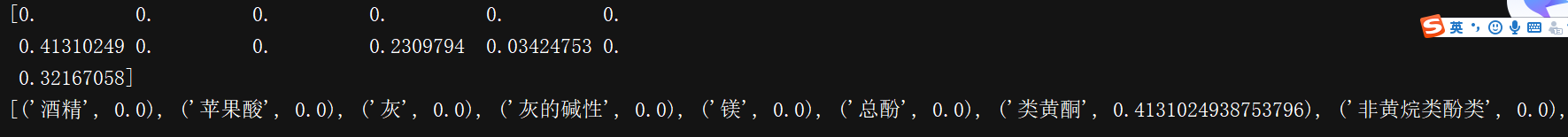2.1.2 random_state & spliter
random_state用来设置分枝中的随机模式的参数，默认None，在高维度时随机性会表现更明显，低维度的数据（比如鸢尾花数据集），随机性几乎不会显现。输入任意整数，会一直长出同一棵树，让模型稳定下来.
splitter也是用来控制决策树中的随机选项的，有两种输入值，输入”best"，决策树在分枝时虽然随机，但是还是会优先选择更重要的特征进行分枝（重要性可以通过属性feature_importances_查看），输入“random"，决策树在分枝时会更加随机，树会因为含有更多的不必要信息而更深更大，并因这些不必要信息而降低对训练集的拟合。这也是防止过拟合的一种方式。当你预测到你的模型会过拟合，用这两个参数来帮助你降低树建成之后过拟合的可能性。当然，树一旦建成，我们依然是使用剪枝参数来防止过拟合.
clf = tree.DecisionTreeClassifier(criterion="entropy",random_state=30,
splitter="random")
clf = clf.fit(xtrain,ytrain)
score = clf.score(xtest,ytest)
print(score)   # 0.9629629629629629

2.1.3 剪枝参数
在不加限制的情况下，一棵决策树会生长到衡量不纯度的指标最优，或者没有更多的特征可用为止。这样的决策树往往会过拟合，这就是说，它会在训练集上表现很好，在测试集上却表现糟糕。我们收集的样本数据不可能和整体的状况完全一致，因此当一棵决策树对训练数据有了过于优秀的解释性，它找出的规则必然包含了训练样本中的噪声，并使它对未知数据的拟合程度不足。
训练集的模型拟合如何？
score_train = tree.DecisionTreeClassifier().fit(xtrain,ytrain).score(xtrain,ytrain)
print(score_train)    # 1

为了让决策树有更好的泛化性，我们要对决策树进行剪枝。剪枝策略对决策树的影响巨大，正确的剪枝策略是优化决策树算法的核心。sklearn为我们提供了不同的剪枝策略。

max_depth
限制树的最大深度，超过设定深度的树枝全部剪掉
这是用得最广泛的剪枝参数，在高维度低样本量时非常有效。决策树多生长一层，对样本量的需求会增加一倍，所以限制树深度能够有效地限制过拟合。在集成算法中也非常实用。实际使用时，建议从=3开始尝试，看看拟合的效果再决定是否增加设定深度
min-samples_leaf & min_samples_split
min_samples_leaf限定，一个节点在分枝后的每个子节点都必须包含至少min_samples_leaf个训练样本，否则分枝就不会发生，或者，分枝会朝着满足每个子节点都包含min_samples_leaf个样本的方向去发生
一般搭配max_depth使用，在回归树中有神奇的效果，可以让模型变得更加平滑。这个参数的数量设置得太小会引起过拟合，设置得太大就会阻止模型学习数据。一般来说，建议从=5开始使用。如果叶节点中含有的样本量变化很大，建议输入浮点数作为样本量的百分比来使用。同时，这个参数可以保证每个叶子的最小尺寸，可以在回归问题中避免低方差，过拟合的叶子节点出现。对于类别不多的分类问题，=1通常就是最佳选择。
min_samples_split限定，一个节点必须要包含至少min_samples_split个训练样本，这个节点才允许被分枝，否则分枝就不会发生。

xtrain,xtest,ytrain,ytest = train_test_split(wine.data,wine.target,test_size=0.3)
clf = tree.DecisionTreeClassifier(criterion="entropy",
random_state=30,
splitter="random",
max_depth= 3,
min_samples_leaf=10,
min_samples_split=20
)
clf = clf.fit(xtrain,ytrain)
test_score = clf.score(xtest,ytest)
print(test_score)   # 0.8888888888888888
train_score = clf.score(xtrain,ytrain)
print(train_score)  # 0.8870967741935484


max_features  & min_impurity_decrease
max_features限制分枝时考虑的特征个数，超过限制个数的特征都会被舍弃。和max_depth异曲同工，
max_features是用来限制高维度数据的过拟合的剪枝参数，但其方法比较暴力，是直接限制可以使用的特征数量而强行使决策树停下的参数，在不知道决策树中的各个特征的重要性的情况下，强行设定这个参数可能会导致模型学习不足。如果希望通过降维的方式防止过拟合，建议使用PCA，ICA或者特征选择模块中的降维算法。
min_impurity_decrease限制信息增益的大小，信息增益小于设定数值的分枝不会发生。这是在0.19版本中更新的功能，在0.19版本之前时使用min_impurity_split。
确认最优的剪枝参数
那具体怎么来确定每个参数填写什么值呢？这时候，我们就要使用确定超参数的曲线来进行判断了，继续使用我们已经训练好的决策树模型clf。超参数的学习曲线，是一条以超参数的取值为横坐标，模型的度量指标为纵坐标的曲线，它是用来衡量不同超参数取值下模型的表现的线。在我们建好的决策树里，我们的模型度量指标就是score。

2.1.4 目标权重参数

class_weight & min_weight_fraction_leaf
完成样本标签平衡的参数。样本不平衡是指在一组数据集中，标签的一类天生占有很大的比例。比如说，在银行要判断“一个办了信用卡的人是否会违约”，就是是vs否（1%：99%）的比例。这种分类状况下，即便模型什么也不做，全把结果预测成“否”，正确率也能有99%。因此我们要使用class_weight参数对样本标签进行一定的均衡，给少量的标签更多的权重，让模型更偏向少数类，向捕获少数类的方向建模。该参数默认None，此模式表示自动给与数据集中的所有标签相同的权重。
有了权重之后，样本量就不再是单纯地记录数目，而是受输入的权重影响了，因此这时候剪枝，就需要搭配min_weight_fraction_leaf这个基于权重的剪枝参数来使用。另请注意，基于权重的剪枝参数（例如min_weight_fraction_leaf）将比不知道样本权重的标准（比如min_samples_leaf）更少偏向主导类。如果样本是加权的，则使用基于权重的预修剪标准来更容易优化树结构，这确保叶节点至少包含样本权重的总和的一小部分。

2.2 重要属性和接口
属性是在模型训练之后，能够调用查看的模型的各种性质。对决策树来说，最重要的feature_importances_，能够查看各个特征对模型的重要性。
sklearn中许多算法的接口都是相似的，比如说我们之前已经用到的fit和score，几乎对每个算法都可以使用。除了这两个接口之外，决策树最常用的接口还有apply和predict。apply中输入测试集返回每个测试样本所在的叶子节点的索引，predict输入测试集返回每个测试样本的标签。返回的内容一目了然并且非常容易，大家感兴趣可以自己下去试试看。
在这里不得不提的是，**所有接口中要求输入X_train和X_test的部分，输入的特征矩阵必须至少是一个二维矩阵。sklearn不接受任何一维矩阵作为特征矩阵被输入。**如果你的数据的确只有一个特征，那必须用reshape(-1,1)来给矩阵增维；如果你的数据只有一个特征和一个样本，使用reshape(1,-1)来给你的数据增维。
# apply返回每个测试集样本所在的叶子节点的索引
print(clf.apply(xtest))
# predict返回每个测试集样本的分类、回归结果
print(clf.predict(xtest))

2.3 小总结：
至此，我们已经写完了分类树DecisionTreeClassifier和决策树绘图的所有基础。我们讲解了决策树的基本流程，分类树的八个参数，一个属性，四个接口，以及绘图所用的代码。
八个参数：Criterion，两个随机参数（random_state, splitter），五个剪枝参数（max_depth, min_samples_split, min_samples_leaf, max_feature, min_impurity_decrease）
一个属性：feature_importances_
四个接口：fit, score, apply, predict
3 回归树DecisionTreeRegressor
class sklearn.tree.DecisionTreeRegressor(criterion=’mse’, splitter=’best’, max_depth=None, min_samples_split=2, min_samples_leaf=1, min_weight_fraction_leaf=0.0, max_features=None, random_state=None, max_leaf_nodes=None, min_impurity_decrease=0.0, min_impurity_split=None, presort=False)

几乎所有参数，属性及接口都和分类树一模一样。需要注意的是，在回归树中，没有标签分布是否均衡的问题，因此没有class_weight这样的参数。
3.1 重要参数、属性以及接口

criterion
回归树衡量分枝质量的指标，支持的标准有三种：

输入"mse"使用均方误差mean squared error(MSE)，父节点和叶子节点之间的均方误差特征选择的标准，这种方法通过使用叶子节点的均值来最小化L2损失。
输入“friedman_mse”使用费尔德曼均方误差，这种指标使用弗里德曼针对潜在分枝中的问题改进后的均方误差。
输入"mae"使用绝对平均误差MAE（mean absolute error），这种指标使用叶节点的中值

属性中最重要的依然是feature_importances_，接口依然是apply, fit, predict, score最核心。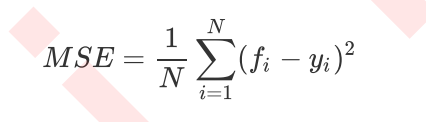其中N是样本数量，i是每一个数据样本，fi是模型回归出的数值，yi是样本点i实际的数值标签。所以MSE的本质，其实是样本真实数据与回归结果的差异。在回归树中，MSE不只是我们的分枝质量衡量指标，也是我们最常用的衡量回归树回归质量的指标，当我们在使用交叉验证，或者其他方式获取回归树的结果时，我们往往选择均方误差作为我们的评估（在分类树中这个指标是score代表的预测准确率）。在回归中，我们追求的是，MSE越小越好。
然而，回归树的接口score返回的是R平方，并不是MSE。R平方被定义如下：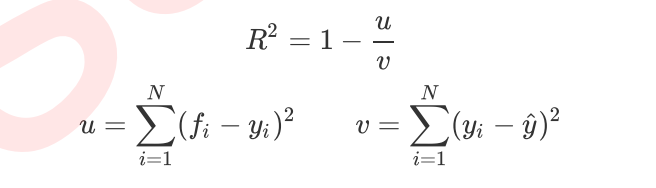其中u是残差平方和（MSE * N），v是总平方和，N是样本数量，i是每一个数据样本，fi是模型回归出的数值，yi是样本点i实际的数值标签。y帽是真实数值标签的平均数。R平方可以为正为负（如果模型的残差平方和远远大于模型的总平方和，模型非常糟糕，R平方就会为负），而均方误差永远为正。
值得一提的是，虽然均方误差永远为正，但是sklearn当中使用均方误差作为评判标准时，却是计算”负均方误差“（neg_mean_squared_error）。这是因为sklearn在计算模型评估指标的时候，会考虑指标本身的性质，均方误差本身是一种误差，所以被sklearn划分为模型的一种损失(loss)，因此在sklearn当中，都以负数表示。真正的均方误差MSE的数值，其实就是neg_mean_squared_error去掉负号的数字。

示例：
交叉验证cross_val_score的用法

from sklearn.datasets import load_boston
from sklearn.tree import DecisionTreeRegressor
from sklearn.model_selection import cross_val_score

reg = DecisionTreeRegressor(random_state=1)
result = cross_val_score(reg,boston.data,boston.target,cv=10,scoring="neg_mean_squared_error")
print(result)
# 交叉验证cross_val_score的用法

交叉验证是用来观察模型的稳定性的一种方法，我们将数据划分为n份，依次使用其中一份作为测试集，其他n-1份作为训练集，多次计算模型的精确性来评估模型的平均准确程度。训练集和测试集的划分会干扰模型的结果，因此用交叉验证n次的结果求出的平均值，是对模型效果的一个更好的度量。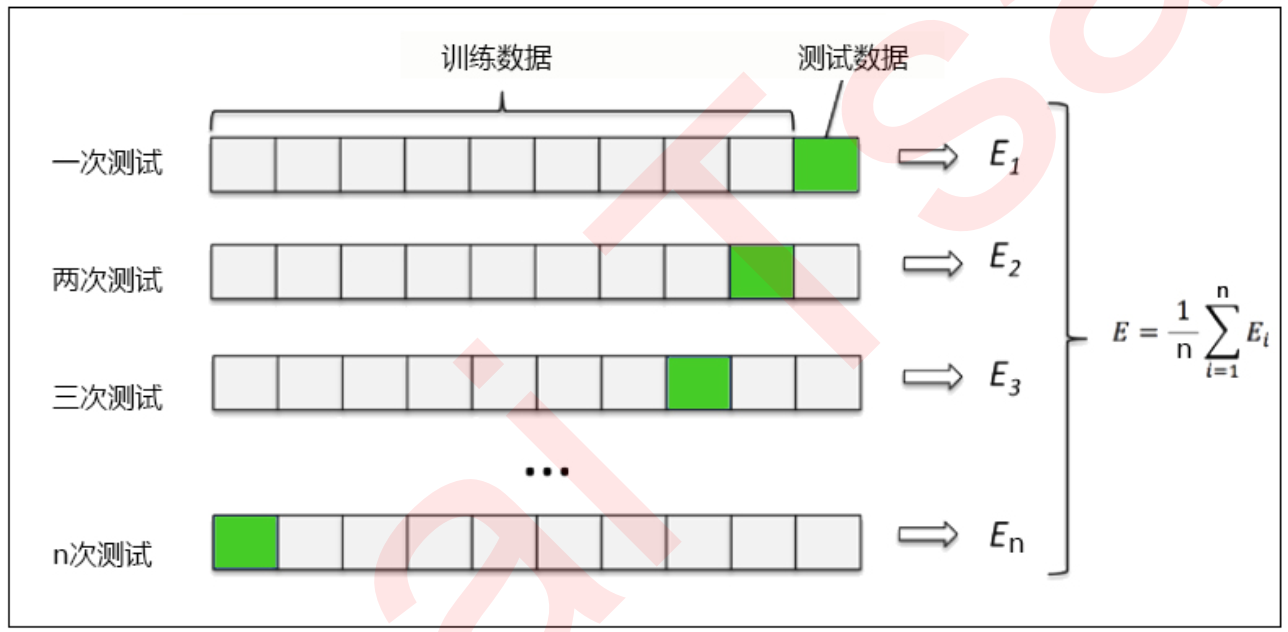3.2 示例：一维回归的图像绘制
我们用回归树来拟合正弦曲线，并添加一些噪声来观察回归树的表现。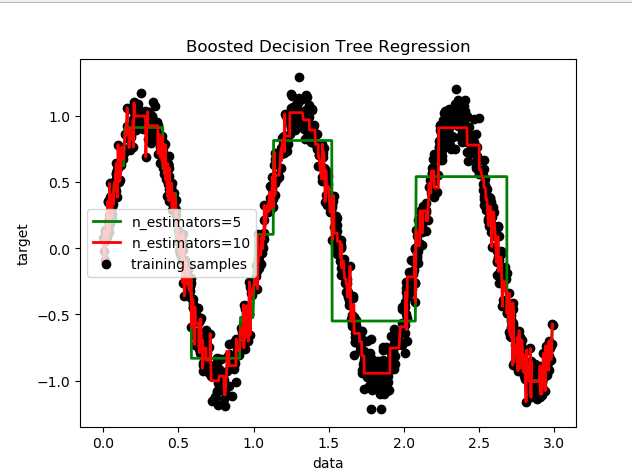# 了解降维函数ravel()的用法
np.random.seed(123)
a = np.random.random((2,2))
b = a.ravel()
print(a)
print(type(a))   # <class 'numpy.ndarray'>
print(a.shape)   # (2, 2)
print(b)
print(type(b))   # <class 'numpy.ndarray'>
print(b.shape)   # (4,)

# 1 导包
import numpy as np
import matplotlib.pyplot as plt
from sklearn.tree import DecisionTreeRegressor

# 2 创建伪数据
rng = np.random.RandomState(1)
x = np.sort(3*rng.rand(1000,1),axis=0)
print(x)
print(x.shape)
y = np.sin(6 * x).ravel() + rng.normal(0,0.1,x.shape)
print(y)
print(y.shape)

# 3. 拟合模型
reg_1 = DecisionTreeRegressor(max_depth=5).fit(x,y)
reg_2 = DecisionTreeRegressor(max_depth=10).fit(x,y)

# 4. predict
y_1 = reg_1.predict(x)
y_2 = reg_2.predict(x)

# 5. Plot the results
plt.figure()
plt.scatter(x, y, c="k", label="training samples")
plt.plot(x, y_1, c="g", label="n_estimators=5", linewidth=2)
plt.plot(x, y_2, c="r", label="n_estimators=10", linewidth=2)
plt.xlabel("data")
plt.ylabel("target")
plt.title("Boosted Decision Tree Regression")
plt.legend()
plt.show()

可见，回归树学习了近似正弦曲线的局部线性回归。我们可以看到，如果树的最大深度（由max_depth参数控制）设置得太高，则决策树学习得太精细，它从训练数据中学了很多细节，包括噪声得呈现，从而使模型偏离真实的正弦曲线，形成过拟合。
4 示例：泰坦尼克号幸存者的预测
4.1 数据总览

导包

# 1. import module
import pandas as pd
from sklearn.tree import DecisionTreeClassifier
from sklearn.model_selection import train_test_split
from sklearn.model_selection import cross_val_score
from sklearn.model_selection import GridSearchCV
import matplotlib.pyplot as plt
import seaborn as sns

sns.set_style('whitegrid')
pd.set_option('display.width',None)
pd.set_option('display.max_rows', None)


导数据

# 2. import data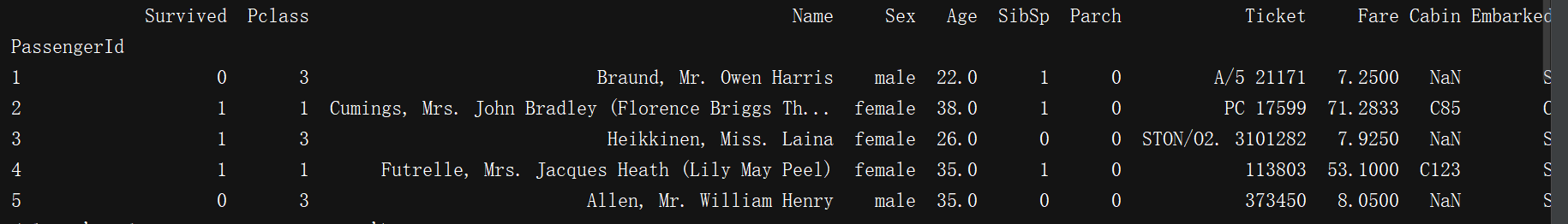查看缺失值信息
print(train.info())
print("-"*40)
print(test.info())

<class 'pandas.core.frame.DataFrame'>
Int64Index: 891 entries, 1 to 891
Data columns (total 11 columns):
Survived    891 non-null int64
Pclass      891 non-null int64
Name        891 non-null object
Sex         891 non-null object
Age         714 non-null float64
SibSp       891 non-null int64
Parch       891 non-null int64
Ticket      891 non-null object
Fare        891 non-null float64
Cabin       204 non-null object
Embarked    889 non-null object
dtypes: float64(2), int64(4), object(5)
memory usage: 83.5+ KB
None
----------------------------------------
<class 'pandas.core.frame.DataFrame'>
Int64Index: 418 entries, 892 to 1309
Data columns (total 10 columns):
Pclass      418 non-null int64
Name        418 non-null object
Sex         418 non-null object
Age         332 non-null float64
SibSp       418 non-null int64
Parch       418 non-null int64
Ticket      418 non-null object
Fare        417 non-null float64
Cabin       91 non-null object
Embarked    418 non-null object
dtypes: float64(2), int64(3), object(5)
memory usage: 35.9+ KB
None

可知：Age，Cabin 缺失值较多，Embarked，Fare 缺失较少
绘制存货比例：
train['Survived'].value_counts().plot.pie(autopct = '%1.2f%%')
plt.show()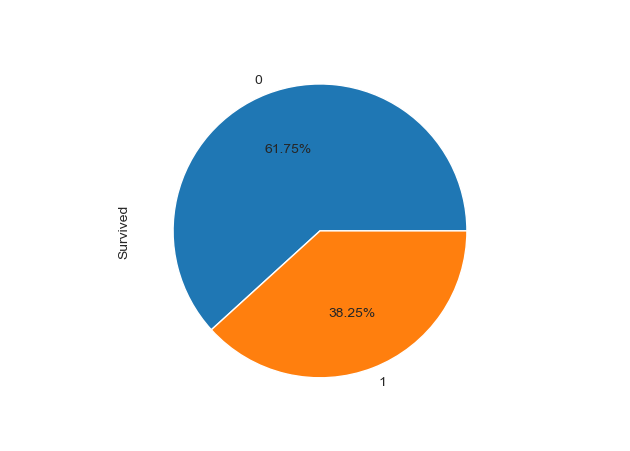4.2 缺失值处理方法
对数据进行分析的时候要注意其中是否有缺失值。
print(data.isnull())   ### 查看data是否有缺失值（空值），False无缺失值
pritn(data.notnull())  ### 查看data是否有缺失值（空值），True无缺失值

一些机器学习算法能够处理缺失值，比如神经网络，一些则不能。对于缺失值，一般有以下几种处理方法：
（1）如果数据集很多，但有很少的缺失值，可以删掉带缺失值的行；
（2）如果该属性相对学习来说不是很重要，可以对缺失值赋均值或者众数。比如在哪儿上船Embarked这一属性（共有三个上船地点），缺失俩值，可以用众数赋值
（3）对于标称属性，可以赋一个代表缺失的值，比如‘U0’。因为缺失本身也可能代表着一些隐含信息。比如船舱号Cabin这一属性，缺失可能代表并没有船舱。
#replace missing value with U0
train_data['Cabin'] = train_data.Cabin.fillna('U0') # train_data.Cabin[train_data.Cabin.isnull()]='U0'

（4）使用回归 随机森林等模型来预测缺失属性的值。因为Age在该数据集里是一个相当重要的特征（先对Age进行分析即可得知），所以保证一定的缺失值填充准确率是非常重要的，对结果也会产生较大影响。一般情况下，会使用数据完整的条目作为模型的训练集，以此来预测缺失值。对于当前的这个数据，可以使用随机森林来预测也可以使用线性回归预测。这里使用随机森林预测模型，选取数据集中的数值属性作为特征（因为sklearn的模型只能处理数值属性，所以这里先仅选取数值特征，但在实际的应用中需要将非数值特征转换为数值特征）
from sklearn.ensemble import RandomForestRegressor

#choose training data to predict age
age_df = train_data[['Age','Survived','Fare', 'Parch', 'SibSp', 'Pclass']]
age_df_notnull = age_df.loc[(train_data['Age'].notnull())]
age_df_isnull = age_df.loc[(train_data['Age'].isnull())]
X = age_df_notnull.values[:,1:]
Y = age_df_notnull.values[:,0]
# use RandomForestRegression to train data
RFR = RandomForestRegressor(n_estimators=1000, n_jobs=-1)
RFR.fit(X,Y)
predictAges = RFR.predict(age_df_isnull.values[:,1:])
train_data.loc[train_data['Age'].isnull(), ['Age']]= predictAges

# 训练集缺失值处理方式
train['Cabin'] = train['Cabin'].fillna('U0')   # Cabin属性缺失值以'U0'代替
train["Age"] = train["Age"].fillna(train["Age"].mean())  # Age属性缺失值以均值代替
train = train.dropna()     # Embarked属性缺失值直接删除    统一删除
print(train.info())

缺失数据处理后的DataFram：
<class 'pandas.core.frame.DataFrame'>
Int64Index: 889 entries, 1 to 891
Data columns (total 11 columns):
Survived    889 non-null int64
Pclass      889 non-null int64
Name        889 non-null object
Sex         889 non-null object
Age         889 non-null float64
SibSp       889 non-null int64
Parch       889 non-null int64
Ticket      889 non-null object
Fare        889 non-null float64
Cabin       889 non-null object
Embarked    889 non-null object
dtypes: float64(2), int64(4), object(5)
memory usage: 83.3+ KB
None

4.3 分析数据关系
(1) 性别与是否生存的关系 Sex
print(train.groupby(["Sex","Survived"])["Survived"].count())   # 加["Survived"]是因为只想统计"Survived"字段
print(train[['Sex','Survived']].groupby(['Sex']).mean())   # train[['Sex','Survived']]道理一致
train[['Sex','Survived']].groupby(['Sex']).mean().plot.bar()
plt.show()

Sex     Survived
female  0            81
1           231
male    0           468
1           109
Name: Survived, dtype: int64
Survived
Sex
female  0.740385
male    0.188908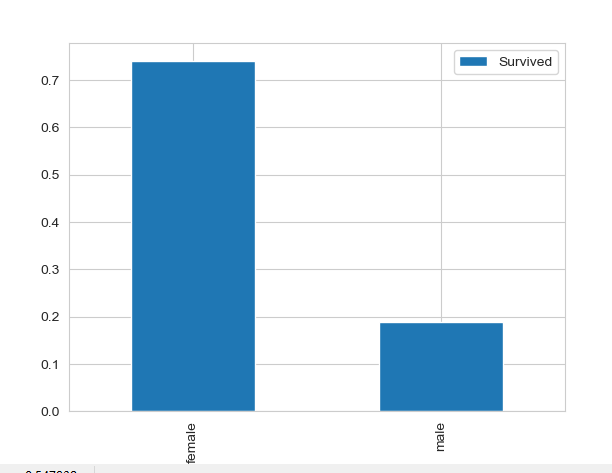注：
print(train.groupby(["Sex","Survived"]).count())

Pclass  Name  Age  SibSp  Parch  Ticket  Fare  Cabin  Embarked
Sex    Survived
female 0             81    81   81     81     81      81    81     81        81
1            231   231  231    231    231     231   231    231       231
male   0            468   468  468    468    468     468   468    468       468
1            109   109  109    109    109     109   109    109       109

(2) 船舱等级和生存与否的关系 Pclass
print(train.groupby(["Pclass","Survived"])['Pclass'].count())
# print(train[['Pclass','Survived']].groupby(['Pclass']).mean())
print(train.groupby(['Pclass'])['Survived'].mean())
train.groupby(['Pclass'])['Survived'].mean().plot.bar()
plt.show()

Pclass  Survived
1       0            80
1           134
2       0            97
1            87
3       0           372
1           119
Name: Pclass, dtype: int64
Pclass
1    0.626168
2    0.472826
3    0.242363
Name: Survived, dtype: float64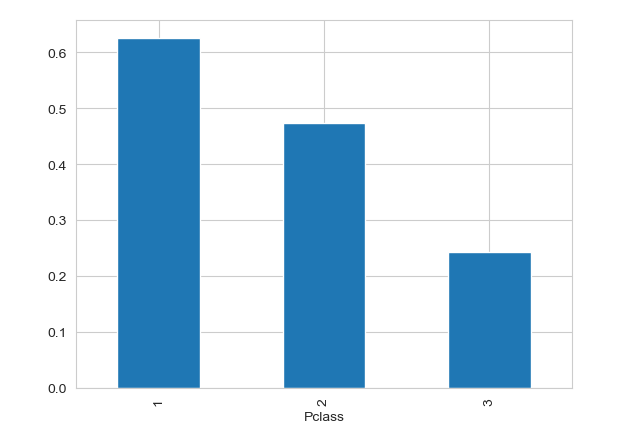sns.countplot(x="Pclass", hue="Survived", data=train)
plt.show()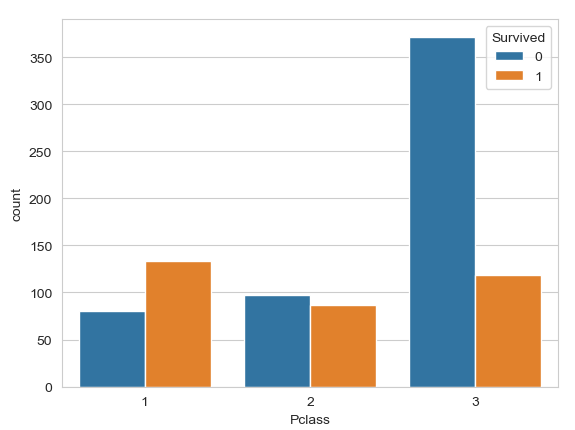train[['Sex','Pclass','Survived']].groupby(['Pclass','Sex']).mean().plot.bar()
print(train[['Sex','Pclass','Survived']].groupby(['Pclass','Sex']).mean())
plt.show()

Survived
Pclass Sex
1      female  0.967391
male    0.368852
2      female  0.921053
male    0.157407
3      female  0.500000
male    0.135447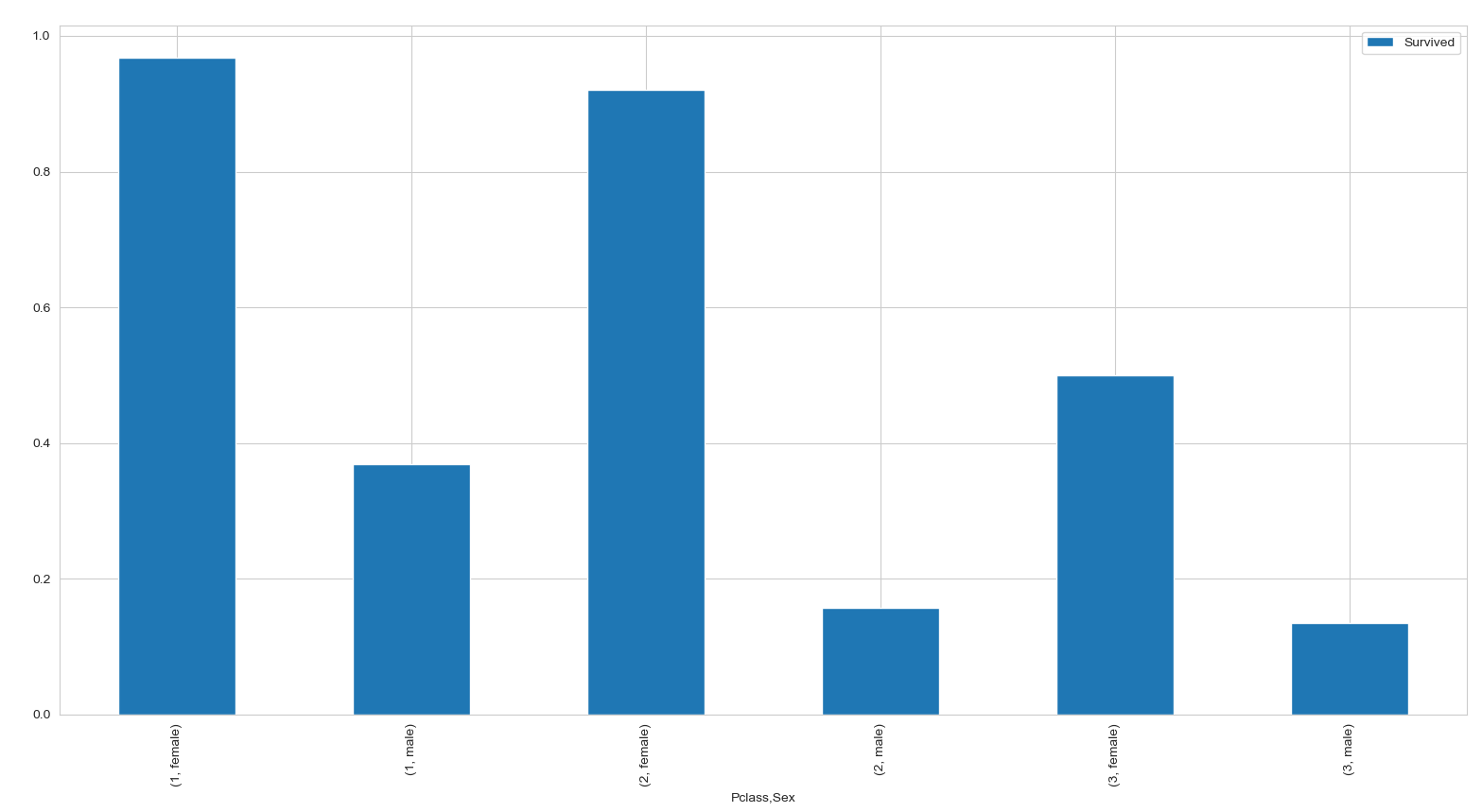不过，无法忽视的是，不同等级的生还率还是有一定区别的。
(3) 年龄与存活与否的关系 Age
分别分析不同等级船舱和不同性别下的年龄分布和生存的关系：
fig, ax = plt.subplots(1, 2, figsize = (18, 8))
sns.violinplot("Pclass", "Age", hue="Survived", data=train, split=True, ax=ax)
ax.set_title('Pclass and Age vs Survived')
ax.set_yticks(range(0, 110, 10))

sns.violinplot("Sex", "Age", hue="Survived", data=train, split=True, ax=ax)
ax.set_title('Sex and Age vs Survived')
ax.set_yticks(range(0, 110, 10))

plt.show()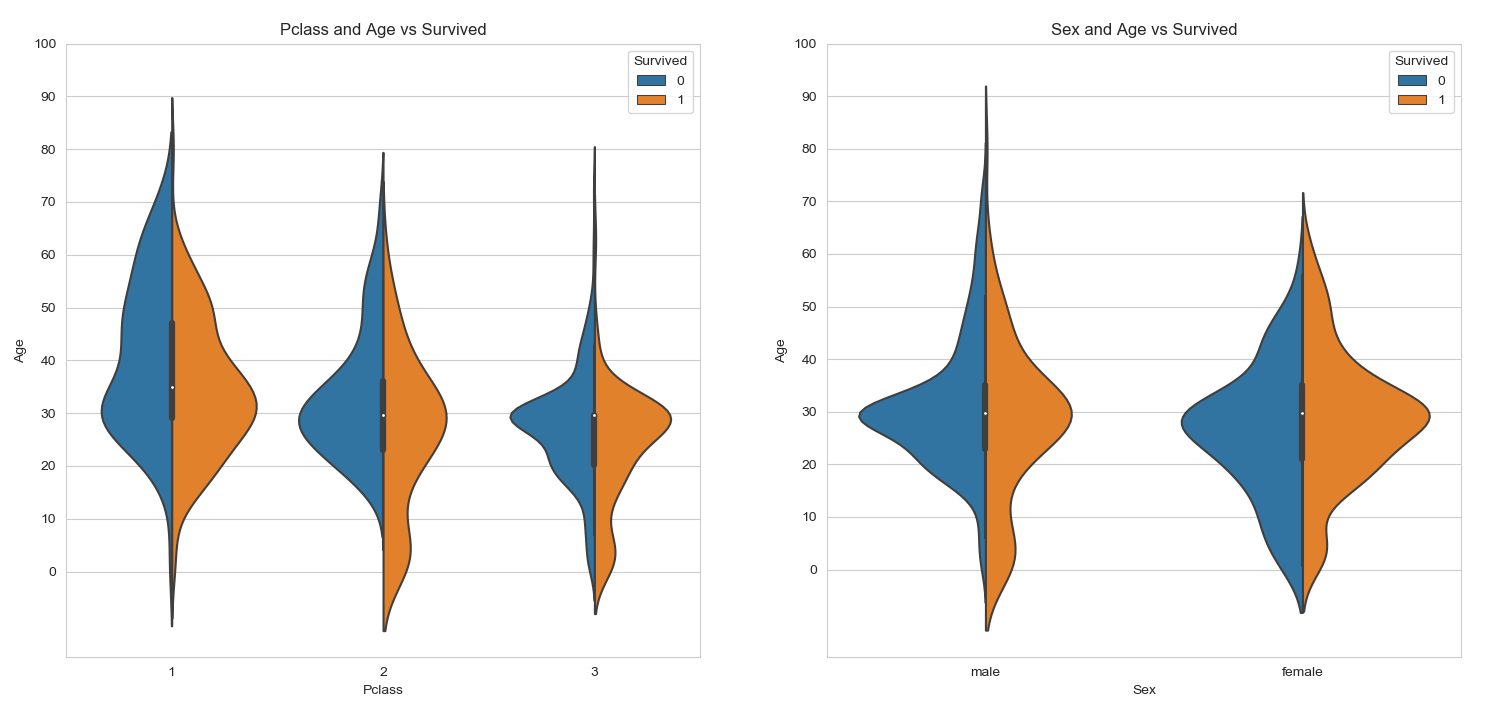分析总体的年龄分布：
plt.figure(figsize=(12,5))
plt.subplot(121)
train['Age'].hist(bins=70)
plt.xlabel('Age')
plt.ylabel('Num')

plt.subplot(122)
train.boxplot(column='Age', showfliers=False)
plt.show()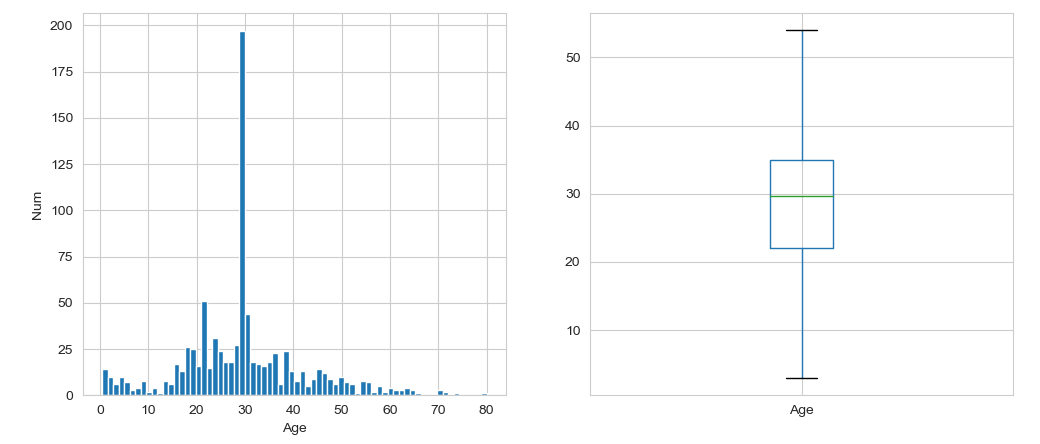不同年龄下的生存和非生存的分布情况：
facet = sns.FacetGrid(train, hue="Survived",aspect=4)
facet.set(xlim=(0, train['Age'].max()))
plt.show()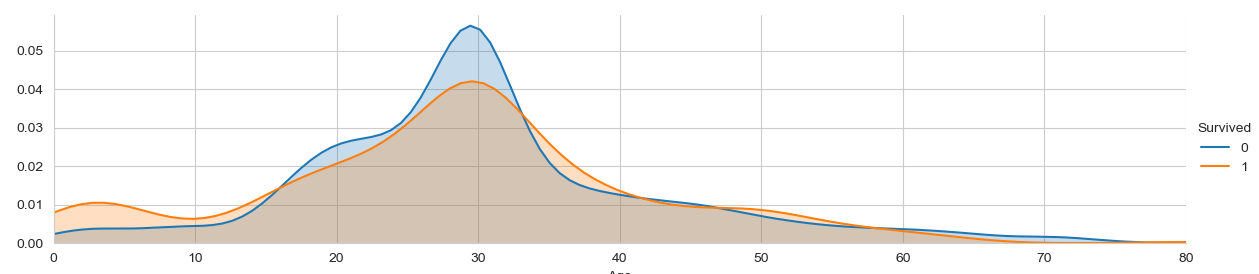不同年龄下的平均生存率：
# average survived passengers by age
fig, axis1 = plt.subplots(1,1,figsize=(18,4))
train["Age_int"] = train["Age"].astype(int)
average_age = train[["Age_int", "Survived"]].groupby(['Age_int'],as_index=False).mean()
sns.barplot(x='Age_int', y='Survived', data=average_age)
plt.show()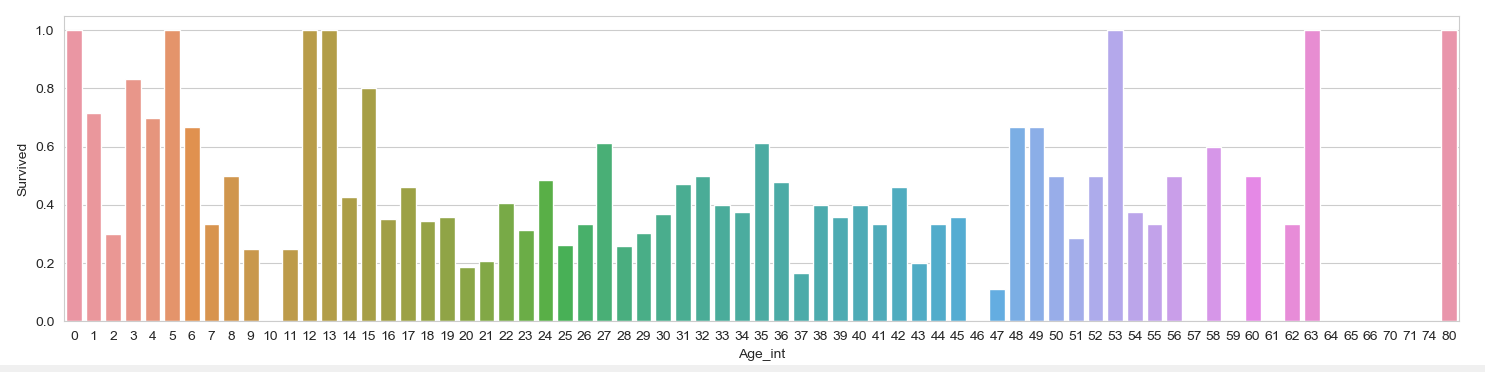print(train['Age'].describe())

count    889.000000
mean      29.653446
std       12.968366
min        0.420000
25%       22.000000
50%       29.699118
75%       35.000000
max       80.000000
Name: Age, dtype: float64


样本有889，平均年龄约为30岁，标准差13岁，最小年龄为0.42，最大年龄80.
按照年龄，将乘客划分为儿童、少年、成年和老年，分析四个群体的生还情况：
bins = [0, 12, 18, 65, 100]
train['Age_group'] = pd.cut(train['Age'], bins)
by_age = train.groupby('Age_group')['Survived'].mean()
print(by_age)
by_age.plot(kind = 'bar')
plt.show()

Age_group
(0, 12]      0.579710
(12, 18]     0.428571
(18, 65]     0.362534
(65, 100]    0.125000
Name: Survived, dtype: float64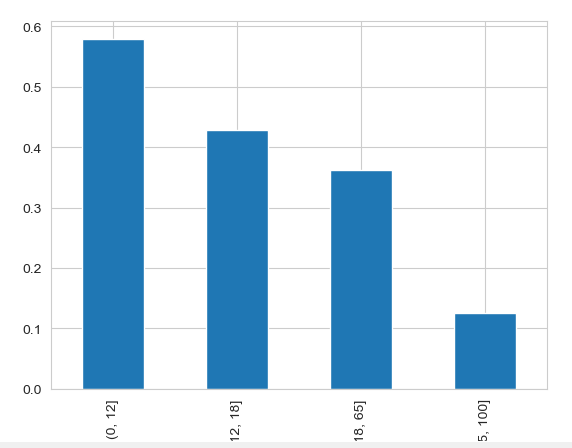(4) 称呼与存活与否的关系 Name
train['Title'] = train['Name'].str.extract(' ([A-Za-z]+)\.', expand=False)
aa = pd.crosstab(train['Title'], train['Sex'])
print(aa)

Sex       female  male
Title
Capt           0     1
Col            0     2
Countess       1     0
Don            0     1
Dr             1     6
Jonkheer       0     1
Major          0     2
Master         0    40
Miss         181     0
Mlle           2     0
Mme            1     0
Mr             0   517
Mrs          124     0
Ms             1     0
Rev            0     6
Sir            0     1

观察不同称呼与生存率的关系：
train[['Title','Survived']].groupby(['Title']).mean().plot.bar()
plt.show()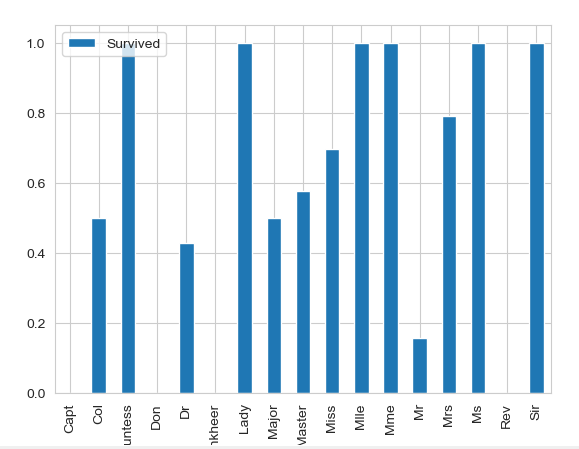同时，对于名字，我们还可以观察名字长度和生存率之间存在关系的可能：
fig, axis1 = plt.subplots(1,1,figsize=(18,4))
train['Name_length'] = train['Name'].apply(len)
name_length = train[['Name_length','Survived']].groupby(['Name_length'],as_index=False).mean()
sns.barplot(x='Name_length', y='Survived', data=name_length)
plt.show()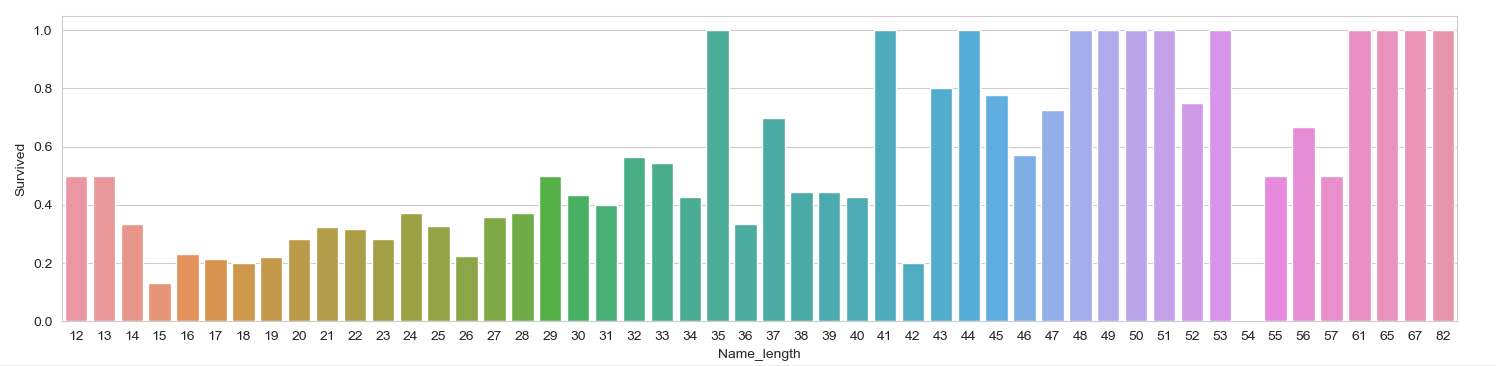从上面的图片可以看出，名字长度和生存与否确实也存在一定的相关性。
(5) 有无兄弟姐妹和存活与否的关系 SibSp
# 将数据分为有兄弟姐妹的和没有兄弟姐妹的两组：
sibsp_df = train[train['SibSp'] != 0]
no_sibsp_df = train[train['SibSp'] == 0]
plt.figure(figsize=(10,5))
plt.subplot(121)
sibsp_df['Survived'].value_counts().plot.pie(labels=['No Survived', 'Survived'], autopct = '%1.1f%%')
plt.xlabel('sibsp')
plt.subplot(122)
no_sibsp_df['Survived'].value_counts().plot.pie(labels=['No Survived', 'Survived'], autopct = '%1.1f%%')
plt.xlabel('no_sibsp')

plt.show()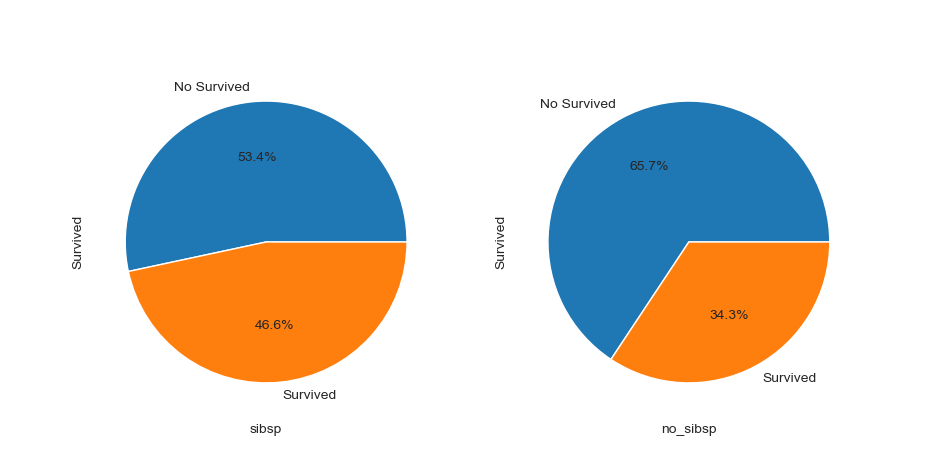(6) 有无父母子女和存活与否的关系 Parch
和有无兄弟姐妹一样，同样分析可以得到：
parch_df = train[train['Parch'] != 0]
no_parch_df = train[train['Parch'] == 0]
plt.figure(figsize=(10,5))
plt.subplot(121)
parch_df['Survived'].value_counts().plot.pie(labels=['No Survived', 'Survived'], autopct = '%1.1f%%')
plt.xlabel('parch')
plt.subplot(122)
no_parch_df['Survived'].value_counts().plot.pie(labels=['No Survived', 'Survived'], autopct = '%1.1f%%')
plt.xlabel('no_parch')
plt.show()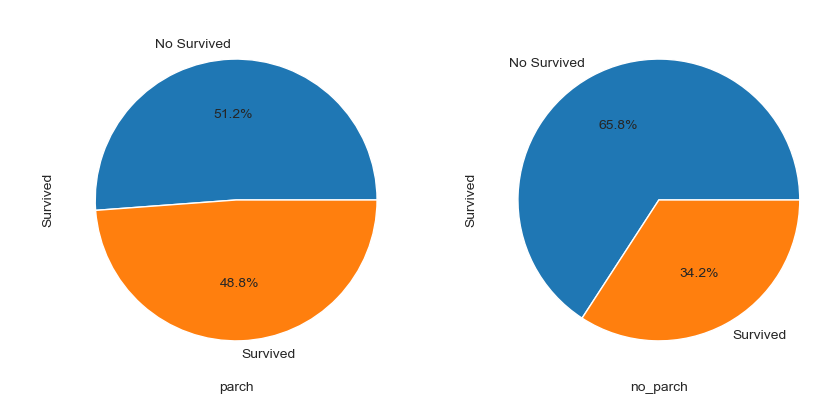(7) 亲友的人数和存活与否的关系 SibSp & Parch
fig,ax=plt.subplots(1,2,figsize=(18,8))
train[['Parch','Survived']].groupby(['Parch']).mean().plot.bar(ax=ax)
ax.set_title('Parch and Survived')
train[['SibSp','Survived']].groupby(['SibSp']).mean().plot.bar(ax=ax)
ax.set_title('SibSp and Survived')
plt.show()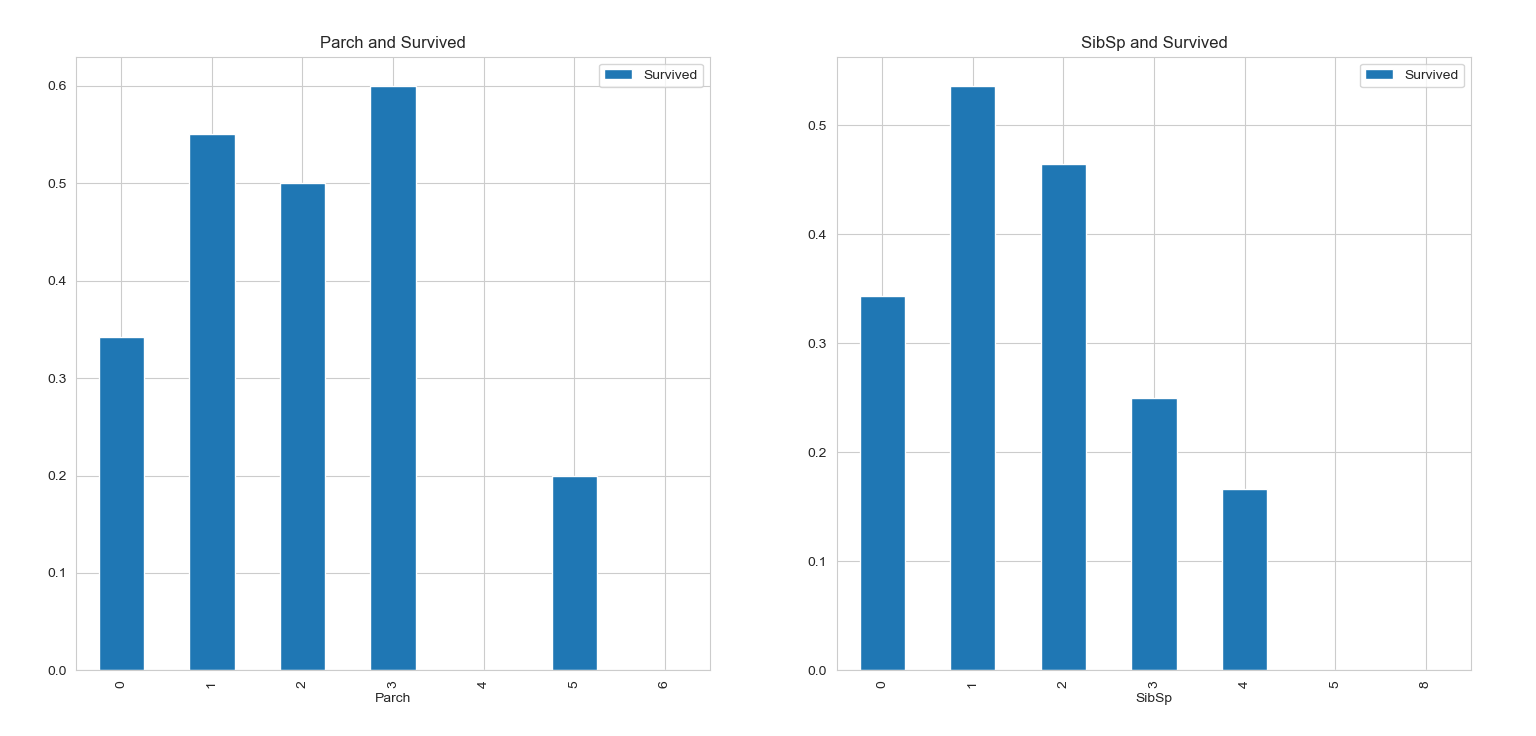train['Family_Size'] = train['Parch'] + train['SibSp'] + 1
train[['Family_Size','Survived']].groupby(['Family_Size']).mean().plot.bar()
plt.show()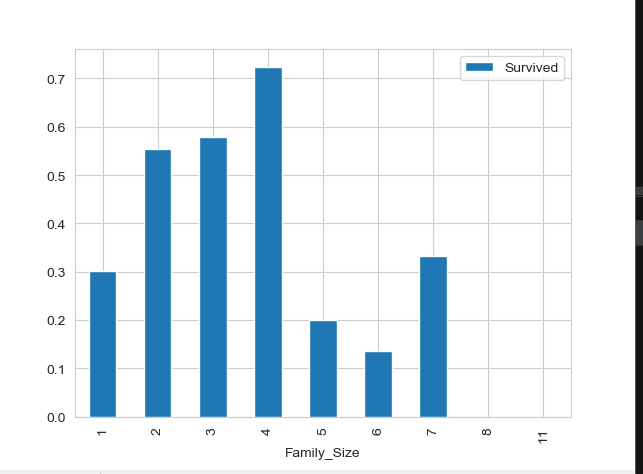从图表中可以看出，若独自一人，那么其存活率比较低；但是如果亲友太多的话，存活率也会很低。
(8) 票价分布和存活与否的关系 Fare
首先绘制票价的分布情况：
plt.figure(figsize=(10,5))
train['Fare'].hist(bins = 70)
train.boxplot(column='Fare', by='Pclass', showfliers=False)
plt.show()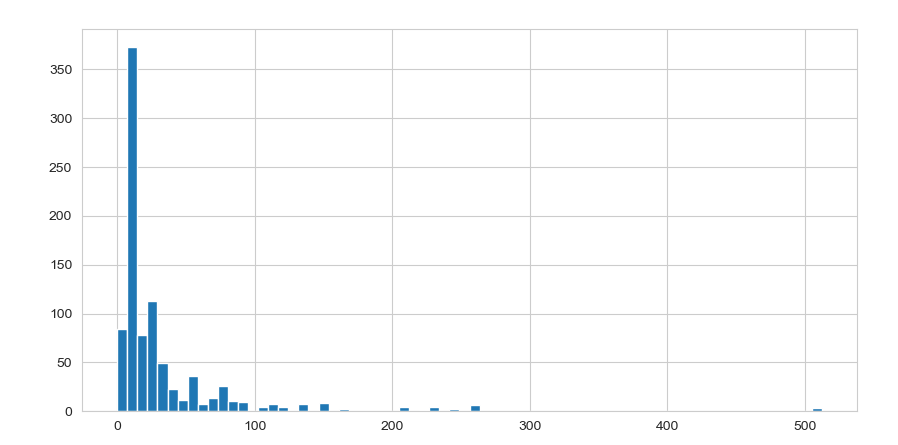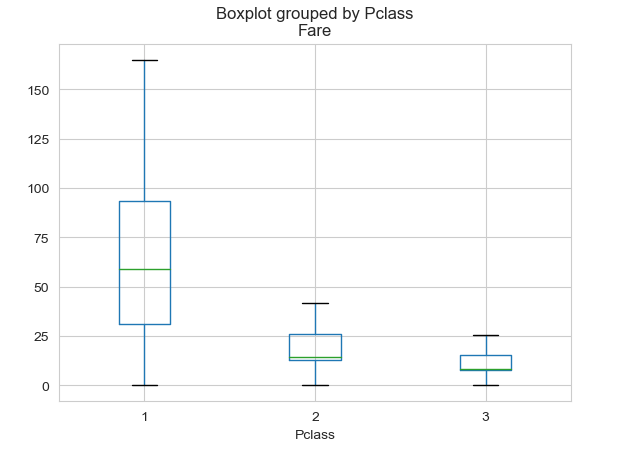train_data['Fare'].describe()

count    891.000000
mean      32.204208
std       49.693429
min        0.000000
25%        7.910400
50%       14.454200
75%       31.000000
max      512.329200
Name: Fare, dtype: float64

绘制生存与否与票价均值和方差的关系：
fare_not_survived = train['Fare'][train['Survived'] == 0]
fare_survived = train['Fare'][train['Survived'] == 1]
average_fare = pd.DataFrame([fare_not_survived.mean(), fare_survived.mean()])
std_fare = pd.DataFrame([fare_not_survived.std(), fare_survived.std()])
average_fare.plot(yerr=std_fare, kind='bar', legend=False)
plt.show()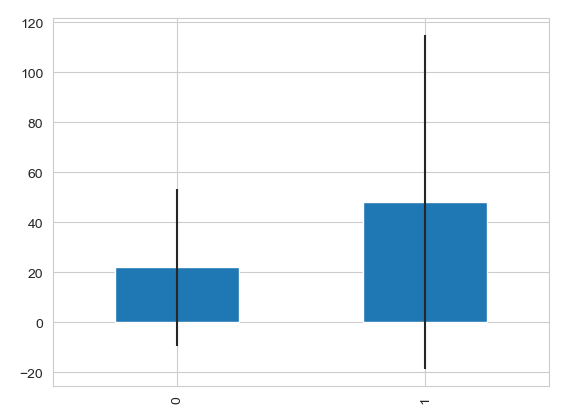由上图标可知，票价与是否生还有一定的相关性，生还者的平均票价要大于未生还者的平均票价。
(9) 船舱类型和存活与否的关系 Cabin
由于船舱的缺失值确实太多，有效值仅仅有204个，很难分析出不同的船舱和存活的关系，所以在做特征工程的时候，可以直接将该组特征丢弃。
当然，这里我们也可以对其进行一下分析，对于缺失的数据都分为一类。
简单地将数据分为是否有Cabin记录作为特征，与生存与否进行分析：
# Replace missing values with "U0"
train.loc[train.Cabin.isnull(), 'Cabin'] = 'U0'
train['Has_Cabin'] = train['Cabin'].apply(lambda x: 0 if x == 'U0' else 1)
train[['Has_Cabin','Survived']].groupby(['Has_Cabin']).mean().plot.bar()
plt.show()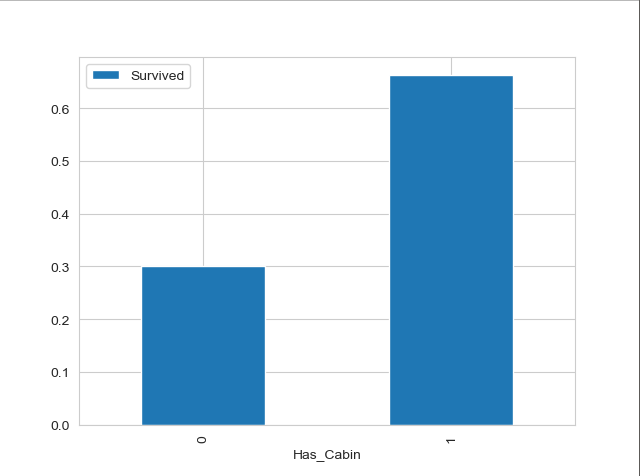对不同类型的船舱进行分析：
import re
# create feature for the alphabetical part of the cabin number
train['CabinLetter'] = train['Cabin'].map(lambda x: re.compile("([a-zA-Z]+)").search(x).group())
# convert the distinct cabin letters with incremental integer values
train['CabinLetter'] = pd.factorize(train['CabinLetter'])
train[['CabinLetter','Survived']].groupby(['CabinLetter']).mean().plot.bar()
plt.show()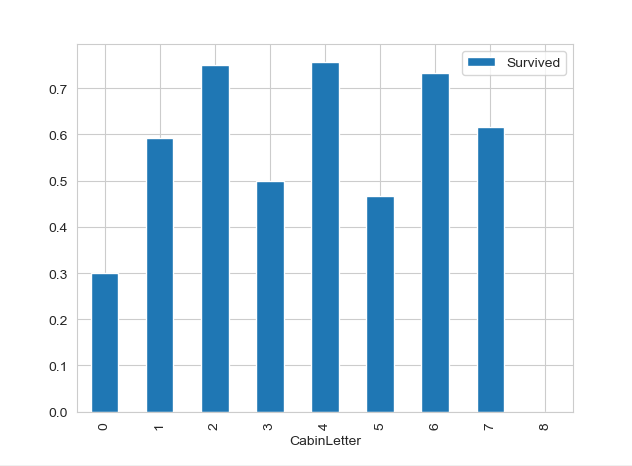可见，不同的船舱生存率也有不同，但是差别不大。所以在处理中，我们可以直接将特征删除。
(10) 港口和存活与否的关系 Embarked
泰坦尼克号从英国的南安普顿港出发，途径法国瑟堡和爱尔兰昆士敦，那么在昆士敦之前上船的人，有可能在瑟堡或昆士敦下船，这些人将不会遇到海难。
sns.countplot('Embarked', hue='Survived', data=train)
plt.title('Embarked and Survived')
plt.show()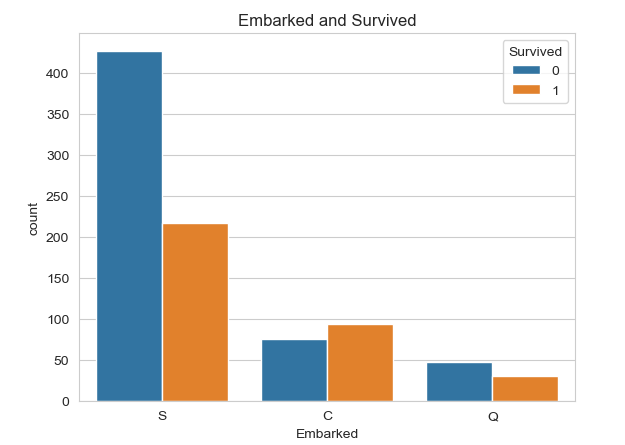sns.factorplot('Embarked', 'Survived', data=train, size=3, aspect=2)
plt.title('Embarked and Survived rate')
plt.show()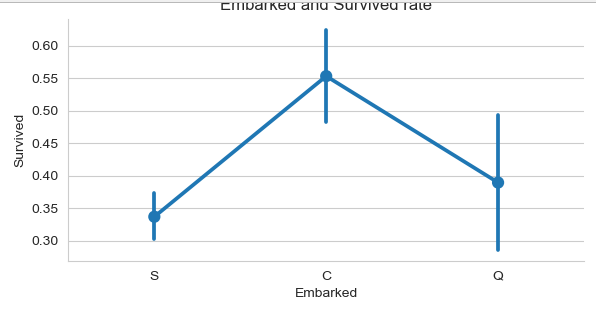由上可以看出，在不同的港口上船，生还率不同，C最高，Q次之，S最低。
以上为所给出的数据特征与生还与否的分析。
据了解，泰坦尼克号上共有2224名乘客。本训练数据只给出了891名乘客的信息，如果该数据集是从总共的2224人中随机选出的，根据中心极限定理，该样本的数据也足够大，那么我们的分析结果就具有代表性；但如果不是随机选取，那么我们的分析结果就可能不太靠谱了。
(11) 其他可能和存活与否有关系的特征
对于数据集中没有给出的特征信息，我们还可以联想其他可能会对模型产生影响的特征因素。如：乘客的国籍、乘客的身高、乘客的体重、乘客是否会游泳、乘客职业等等。
另外还有数据集中没有分析的几个特征：Ticket（船票号）、Cabin（船舱号）,这些因素的不同可能会影响乘客在船中的位置从而影响逃生的顺序。但是船舱号数据缺失，船票号类别大，难以分析规律，所以在后期模型融合的时候，将这些因素交由模型来决定其重要性。
4.4 变量转换
变量转换的目的是将数据转换为适用于模型使用的数据，不同模型接受不同类型的数据，Scikit-learn要求数据都是数字型numeric，所以我们要将一些非数字型的原始数据转换为数字型numeric。
所以下面对数据的转换进行介绍，以在进行特征工程的时候使用。
所有的数据可以分为两类：

1.定量(Quantitative)变量可以以某种方式排序，Age就是一个很好的列子。
2.定性(Qualitative)变量描述了物体的某一（不能被数学表示的）方面，Embarked就是一个例子。

定性(Qualitative)转换：

Dummy Variables
就是类别变量或者二元变量，当qualitative variable是一些频繁出现的几个独立变量时，Dummy Variables比较适合使用。我们以Embarked为例，Embarked只包含三个值’S’,‘C’,‘Q’，我们可以使用下面的代码将其转换为dummies:

embark_dummies  = pd.get_dummies(train['Embarked'])
train = train.join(embark_dummies)
train.drop(['Embarked'], axis=1,inplace=True)

embark_dummies = train[['S', 'C', 'Q']]

Survived  Pclass                                               Name     Sex   Age  SibSp  Parch            Ticket     Fare Cabin  C  Q  S
PassengerId
1                   0       3                            Braund, Mr. Owen Harris    male  22.0      1      0         A/5 21171   7.2500    U0  0  0  1
2                   1       1  Cumings, Mrs. John Bradley (Florence Briggs Th...  female  38.0      1      0          PC 17599  71.2833   C85  1  0  0
3                   1       3                             Heikkinen, Miss. Laina  female  26.0      0      0  STON/O2. 3101282   7.9250    U0  0  0  1
4                   1       1       Futrelle, Mrs. Jacques Heath (Lily May Peel)  female  35.0      1      0            113803  53.1000  C123  0  0  1
5                   0       3                           Allen, Mr. William Henry    male  35.0      0      0            373450   8.0500    U0  0  0  1
S  C  Q
PassengerId
1            1  0  0
2            0  1  0
3            1  0  0
4            1  0  0
5            1  0  0



Factorizing
dummy不好处理Cabin（船舱号）这种标称属性，因为他出现的变量比较多。所以Pandas有一个方法叫做factorize()，它可以创建一些数字，来表示类别变量，对每一个类别映射一个ID，这种映射最后只生成一个特征，不像dummy那样生成多个特征。

import re
# Replace missing values with "U0"
train['Cabin'][train.Cabin.isnull()] = 'U0'
# create feature for the alphabetical part of the cabin number
train['CabinLetter'] = train['Cabin'].map( lambda x : re.compile("([a-zA-Z]+)").search(x).group())
# convert the distinct cabin letters with incremental integer values
train['CabinLetter'] = pd.factorize(train['CabinLetter'])

PassengerId
1    0
2    1
3    0
4    1
5    0

定量(Quantitative)转换：

Scaling
Scaling可以将一个很大范围的数值映射到一个很小的范围(通常是-1 - 1，或则是0 - 1)，很多情况下我们需要将数值做Scaling使其范围大小一样，否则大范围数值特征将会由更高的权重。比如：Age的范围可能只是0-100，而income的范围可能是0-10000000，在某些对数组大小敏感的模型中会影响其结果。

下面对Age进行Scaling：
from sklearn import preprocessing

assert np.size(train_data['Age']) == 891
# StandardScaler will subtract the mean from each value then scale to the unit variance
scaler = preprocessing.StandardScaler()
train_data['Age_scaled'] = scaler.fit_transform(train_data['Age'].values.reshape(-1, 1))

0   -0.558449
1    0.606773
2   -0.267144
3    0.388293
4    0.388293
Name: Age_scaled, dtype: float64



Binning
Binning通过观察“邻居”(即周围的值)将连续数据离散化。存储的值被分布到一些“桶”或“箱“”中，就像直方图的bin将数据划分成几块一样。下面的代码对Fare进行Binning。

# Divide all fares into quartiles
train_data['Fare_bin'] = pd.qcut(train_data['Fare'], 5)

0      (-0.001, 7.854]
1    (39.688, 512.329]
2        (7.854, 10.5]
3    (39.688, 512.329]
4        (7.854, 10.5]
Name: Fare_bin, dtype: category
Categories (5, interval[float64]): [(-0.001, 7.854] < (7.854, 10.5] < (10.5, 21.679] < (21.679, 39.688] < (39.688, 512.329]]


在将数据Bining化后，要么将数据factorize化，要么dummies化。
# qcut() creates a new variable that identifies the quartile range, but we can't use the string
# so either factorize or create dummies from the result

# factorize
train_data['Fare_bin_id'] = pd.factorize(train_data['Fare_bin'])

# dummies
fare_bin_dummies_df = pd.get_dummies(train_data['Fare_bin']).rename(columns=lambda x: 'Fare_' + str(x))
train_data = pd.concat([train_data, fare_bin_dummies_df], axis=1)

4.5. 特征工程
在进行特征工程的时候，我们不仅需要对训练数据进行处理，还需要同时将测试数据同训练数据一起处理，使得二者具有相同的数据类型和数据分布。


展开全文...